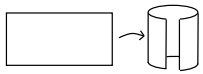Home > A2C > Chapter 8 > Lesson 8.1.3 > Problem8-40

8-40.
1.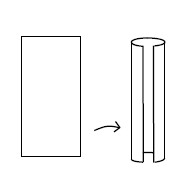Suppose you were to bend two whole sheets of 8 1/2-by-11 paper to form two cylinders (a tall, skinny one and a short, wide one). The volume of a cylinder can be calculated using the formula V = (base area)(height) or V = πr2h. Homework Help ✎

1. Do the two cylinders have the same volume? Justify your answer.

2. Is the result different if you start with-by-paper?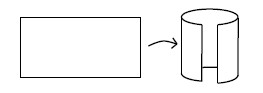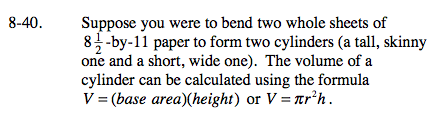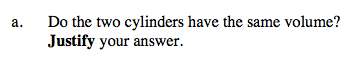Calculate the volume of both cylinders and compare.

Remember, for cylinders:
V = πr2h
C = 2πr
Where V is volume, r is radius, h is height, and C is circumference.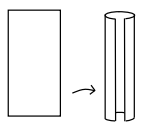For the tall cylinder:

$V=\pi\left(\frac{8\frac{1}{2}}{2\pi}\right)^{2}(11)$

$V=\pi\left(\frac{289}{16\pi^{2}}\right)(11)$

$V=\frac{3179}{16\pi}$

V ≈ 63.24 in.3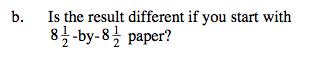See part (a).